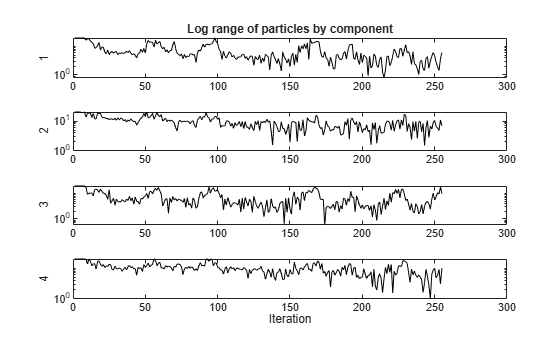# Particle Swarm Output Function

This example shows how to use an output function for `particleswarm`. The output function plots the range that the particles occupy in each dimension.

An output function runs after each iteration of the solver. For syntax details, and for the data available to an output function, see the `particleswarm` options reference pages.

### Custom Plot Function

This output function draws a plot with one line per dimension. Each line represents the range of the particles in the swarm in that dimension. The plot is log-scaled to accommodate wide ranges. If the swarm converges to a single point, then the range of each dimension goes to zero. But if the swarm does not converge to a single point, then the range stays away from zero in some dimensions.

Copy the following code into a file named `pswplotranges.m` on your MATLAB® path. The code sets up `nplot` subplots, where `nplot` is the number of dimensions in the problem.

```function stop = pswplotranges(optimValues,state) stop = false; % This function does not stop the solver switch state case 'init' nplot = size(optimValues.swarm,2); % Number of dimensions for i = 1:nplot % Set up axes for plot subplot(nplot,1,i); tag = sprintf('psoplotrange_var_%g',i); % Set a tag for the subplot semilogy(optimValues.iteration,0,'-k','Tag',tag); % Log-scaled plot ylabel(num2str(i)) end xlabel('Iteration','interp','none'); % Iteration number at the bottom subplot(nplot,1,1) % Title at the top title('Log range of particles by component') setappdata(gcf,'t0',tic); % Set up a timer to plot only when needed case 'iter' nplot = size(optimValues.swarm,2); % Number of dimensions for i = 1:nplot subplot(nplot,1,i); % Calculate the range of the particles at dimension i irange = max(optimValues.swarm(:,i)) - min(optimValues.swarm(:,i)); tag = sprintf('psoplotrange_var_%g',i); plotHandle = findobj(get(gca,'Children'),'Tag',tag); % Get the subplot xdata = plotHandle.XData; % Get the X data from the plot newX = [xdata optimValues.iteration]; % Add the new iteration plotHandle.XData = newX; % Put the X data into the plot ydata = plotHandle.YData; % Get the Y data from the plot newY = [ydata irange]; % Add the new value plotHandle.YData = newY; % Put the Y data into the plot end if toc(getappdata(gcf,'t0')) > 1/30 % If 1/30 s has passed drawnow % Show the plot setappdata(gcf,'t0',tic); % Reset the timer end case 'done' % No cleanup necessary end ```

### Objective Function

The `multirosenbrock` function is a generalization of Rosenbrock's function to any even number of dimensions. It has a global minimum of `0` at the point `[1,1,1,1,...]`.

```function F = multirosenbrock(x) % This function is a multidimensional generalization of Rosenbrock's % function. It operates in a vectorized manner, assuming that x is a matrix % whose rows are the individuals. % Copyright 2014 by The MathWorks, Inc. N = size(x,2); % assumes x is a row vector or 2-D matrix if mod(N,2) % if N is odd error('Input rows must have an even number of elements') end odds = 1:2:N-1; evens = 2:2:N; F = zeros(size(x)); F(:,odds) = 1-x(:,odds); F(:,evens) = 10*(x(:,evens)-x(:,odds).^2); F = sum(F.^2,2); ```

### Set Up and Run Problem

Set the `multirosenbrock` function as the objective function. User four variables. Set a lower bound of `-10` and an upper bound of `10` on each variable.

```fun = @multirosenbrock; nvar = 4; % A 4-D problem lb = -10*ones(nvar,1); % Bounds to help the solver converge ub = -lb; ```

Set options to use the output function.

```options = optimoptions(@particleswarm,'OutputFcn',@pswplotranges); ```

Set the random number generator to get reproducible output. Then call the solver.

```rng default % For reproducibility [x,fval,eflag] = particleswarm(fun,nvar,lb,ub,options) ```
```Optimization ended: relative change in the objective value over the last OPTIONS.MaxStallIterations iterations is less than OPTIONS.FunctionTolerance. x = 0.9964 0.9930 0.9835 0.9681 fval = 3.4935e-04 eflag = 1 ```### Results

The solver returned a point near the optimum `[1,1,1,1]`. But the span of the swarm did not converge to zero.

## Support평가판 신청